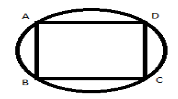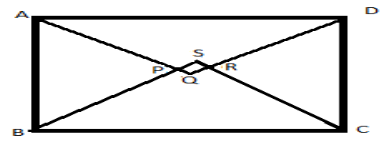QuestionAnswers

# Prove that the quadrilateral formed (if possible) by the internal angle bisectors of any quadrilateral is cyclic.Verified
128.4k+ views
Hint: A quadrilateral is a polygon having four sides and four vertices. A quadrilateral is cyclic when all of its vertices lie on a circle.
A cyclic quadrilateral is given by the following figure:A quadrilateral formed by the internal angle bisectors of a quadrilateral ABCD is PQRS:We need to prove PQRS is cyclic.
A rule of angle equality is, vertically opposite angles are equal.
Angle sum property of a triangle gives the sum of all angles of a triangle = ${180^0}$
To prove the quadrilateral PQRS is cyclic, it is enough to prove that the sum of opposite angles of PQRS is ${180^0}$ . Implies $\angle SPQ + \angle SRQ = {180^0}$

Step 1: Consider a quadrilateral ABCD with internal bisectors AQ, BS, CS, DQ of angles $\angle A$, $\angle B$ , $\angle C$ and $\angle D$ respectively. We need to prove the quadrilateral PQRS formed by these four internal angle bisectors is cyclic.
$\angle SPQ = \angle APB \\ \angle SRQ = \angle DRC \\$
Adding above two formulas we get $\angle SPQ + \angle SRQ = \angle APB + \angle DRC$
As AQ is the angle bisector of $\angle A$ and P is a point on AQ, By angle sum property,
$\angle APB = 180 - (\dfrac{1}{2}\angle A + \dfrac{1}{2}\angle B)$ and $\angle DRC = 180 - (\dfrac{1}{2}\angle D + \dfrac{1}{2}\angle C)$ . Thus,
$\angle SPQ + \angle SRQ = \angle APB + \angle DRC \\ = 180 - (\dfrac{1}{2}\angle A + \dfrac{1}{2}\angle B) + 180 - (\dfrac{1}{2}\angle D + \dfrac{1}{2}\angle C) \\ = 360 - \dfrac{1}{2}(\angle A + \angle B + \angle C + \angle D) \\ = 360 - \dfrac{1}{2}(360) \\ = {180^0} \\$
The sum of opposite angles of quadrilateral PQRS is ${180^0}$ . Thus PQRS is a cyclic quadrilateral.